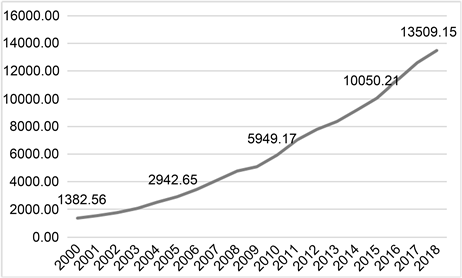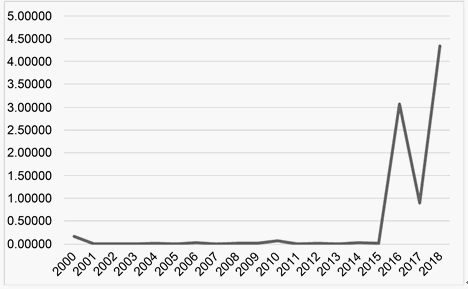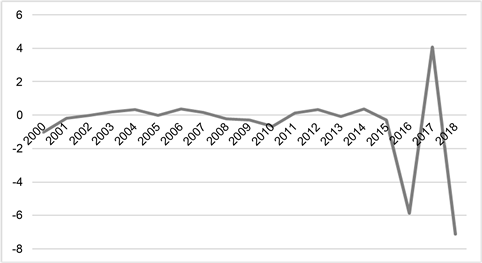# 杭州市GDP数据的统计诊断分析Statistical Diagnosis Analysis of Hangzhou’s GDP Data

DOI: 10.12677/SA.2020.92032, PDF, HTML, XML, 下载: 115  浏览: 253  科研立项经费支持

Abstract: Gross domestic product (GDP) is often recognized as the best indicator to measure the economic situation of a country, which can reflect the economic strength and market size of a country or region. In order to explore the main factors affecting Hangzhou’s GDP, this paper selects several national economic indicators of Hangzhou from 2000 to 2018, and establishes a regression model based on multiple linear regression. Then the stepwise regression method is used to screen variables, and statistical diagnosis is carried out for the regression model. The strong influence points in the model are screened under certain standards. Based on the data deletion model after deleting the strong influence points, the stepwise regression analysis is carried out again, and the conclusion is that the GDP of Hangzhou is closely related to the year-end resident population, fixed asset investment, resident consumption index and total fiscal revenue.

1. 引言

GDP是国民经济核算的核心指标，是反映各地区经济实力和国民生活水平的指标，受到人们的广泛关注，GDP数据质量的提高，对调控宏观经济有很大的促进作用。研究影响GDP变化的主要因素，建立一个可靠合理的模型，有助于找到提高国民经济水平和地区发展的关键因素，对影响GDP的因素进行定量分析和预测具有重要意义，促进GDP不断增长。

2. 数据的收集和杭州市GDP变化的基本情况Figure 1. GDP change of Hangzhou in 2000~2018 (100 million yuan)

3. 模型建立Table 1. Multiple linear regression results

$Y=2.909{X}_{1}+3.702{X}_{2}+0.476{X}_{3}+10.702{X}_{4}+1.352{X}_{5}-8740.869$ (1)

4. 统计诊断

4.1. 影响分析

4.1.1. 基于Cook距离的统计诊断 

$C{D}_{i}=\frac{{\left(\stackrel{^}{\beta }-{\stackrel{^}{\beta }}_{\left(i\right)}\right)}^{\text{T}}{X}^{\text{T}}X\left(\stackrel{^}{\beta }-{\stackrel{^}{\beta }}_{\left(i\right)}\right)}{\left(p+1\right){\stackrel{^}{\sigma }}^{2}}$

4.1.2. 基于W-K统计量的统计诊断 

$W{K}_{i}=\frac{{\stackrel{^}{y}}_{i}-{\stackrel{˜}{y}}_{\left(i\right)}}{{\stackrel{^}{\sigma }}_{\left(i\right)}\sqrt{{h}_{ii}}}=\frac{{\stackrel{˜}{x}}_{i}\left(\stackrel{^}{\beta }-{\stackrel{^}{\beta }}_{\left(i\right)}\right)}{{\stackrel{^}{\sigma }}_{\left(i\right)}\sqrt{{h}_{ii}}}$

$W{K}_{i}=\sqrt{\frac{{h}_{ii}}{1-{h}_{ii}}}{t}_{i}$, ${\left(W{K}_{i}\right)}^{2}=C{D}_{i}\left({X}^{\text{T}}X,{\stackrel{^}{\sigma }}_{\left(i\right)}^{2}\right)$, ${t}_{i}=\frac{{e}_{i}}{{\stackrel{^}{\sigma }}_{\left(i\right)}\sqrt{1-{h}_{ii}}}$

$W{K}_{i}$ 度量了 $\left(\stackrel{^}{\beta },{\stackrel{^}{\sigma }}^{2}\right)$$\left({\stackrel{^}{\beta }}_{\left(i\right)},{\stackrel{^}{\sigma }}_{\left(i\right)}^{2}\right)$ 之间的差异，比Cook距离更加通用，也更容易地被应用到线性模型以外的各种更复杂的统计模型。

4.2. 实例研究Figure 2. Scatter plot of Cook distanceFigure 3. Scatter plot of W-K statisticsTable 2. Summary of strong impact points

5. 模型修正

5.1. 数据删除模型概述

5.1.1. 数据删除模型

${Y}_{\left(i\right)}={X}_{\left(i\right)}\beta +{\epsilon }_{\left(i\right)},{\epsilon }_{\left(i\right)}\sim N\left(0,{\sigma }^{2}{I}_{n-1}\right)$

5.1.2. 数据删除模型的参数估计

${\stackrel{^}{\beta }}_{\left(i\right)}=\stackrel{^}{\beta }-\frac{{\left({X}^{\text{T}}X\right)}^{-1}{\stackrel{˜}{x}}_{i}{e}_{i}}{1-{h}_{ii}},{\stackrel{^}{\sigma }}_{\left(i\right)}^{2}=\frac{n-p-1-{r}_{i}^{2}}{n-p-2}{\stackrel{^}{\sigma }}^{2}$

5.2. 实例研究Table 3. Modified multiple regression resultsTable 4. Stepwise regression results

$Y=14.944{X}_{1}+0.280{X}_{3}+11.622{X}_{4}+1.691{X}_{5}-15326.525$ (2)

6. 结论与建议

  郭芳, 冷洛.国内生产总值影响因素的计量分析[J]. 云南财贸学院学报(社会科学版), 2008, 23(1): 90-92.  文静. 影响国内生产总值的因素分析[J]. 商业文化(下半月), 2011(5): 109.  张卜元, 刘冰冰, 张东旭. 我国国内生产总值影响因素实证分析[J]. 合作经济与科技, 2016(2): 10-11.  程静. 国内生产总值影响因素分析[J]. 经济研究导刊, 2014(7): 7+46.  单翔翔, 严浩坤. 基于多元回归模型分析我国国内生产总值的影响因素[J]. 时代金融, 2018(9): 238-239.  赵深淼, 张英, 刘洋. 北京市国内生产总值影响因素分析[J]. 佳木斯大学学报(自然科学版), 2017, 35(4): 681-683.  黄潇逸. 基于多元线性回归分析的地区生产总值影响因素研究[C]//浙江省地理学会. 浙江省地理学会2018年学术年会暨“城市国际化研究”高峰论坛论文摘要集. 浙江省地理学会: 浙江省地理学会, 2018: 15.  李彦芙. 基于多元线性回归模型的江苏省GDP增长影响因素研究[J]. 特区经济, 2019(4): 84-88.  王璐. 带线性约束的多元线性回归模型的统计诊断[D]: [硕士学位论文]. 南京: 南京理工大学, 2008.  唐年胜，李会琼. 应用回归分析[M]. 北京: 科学出版社, 2014: 97-102, 105-108.  黄守坤. 回归诊断中例外数据点及大影响点的处理[J]. 统计与决策, 2002(6): 47.  赵喜仓, 渠田田, 许鲜欣. 数据删除模型在GDP诊断中的应用[J]. 统计与决策, 2011(10): 8-10.  胡章刚. 线性回归诊断若干问题研究[D]: [硕士学位论文]. 武汉: 武汉科技大学, 2010.  王彤. 线性回归模型的稳健估计及多个异常点诊断方法研究[D]: [博士学位论文]. 西安: 第四军医大学, 2000.